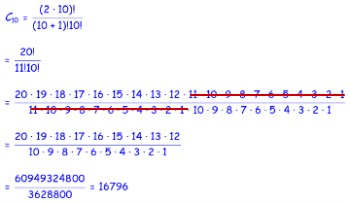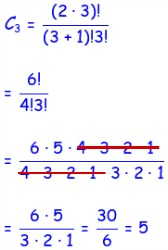# Catalan Numbers: History & Definition

Instructor: Laura Pennington

Laura has taught collegiate mathematics and holds a master's degree in pure mathematics.

This lesson will define the Catalan numbers. We will then review the history of these numbers, including some of the mathematicians and applications that led to the discovery and development of these numbers.

## Catalan Numbers

The Catalan numbers are a fascinating sequence of numbers in mathematics that show up in many different applications. Technically speaking, the nth Catalan number, Cn, is given by the following formula:

• Cn = (2n)! / ((n + 1)!n!), where n! = n ⋅ (n - 1) ⋅ (n - 2) ⋅ … ⋅ 3 ⋅ 2 ⋅ 1

For example, the 10th Catalan number would be found by plugging 10 into the formula for n.We get that C10 = 16796.

Well, sure these numbers sound neat, but we described them as 'fascinating'. Don't worry - they are! You see, these numbers show up in a large variety of applications in combinatorics, or counting processes. For instance, consider the set of integers from 1 to n, or the first n integers.

• {1, 2, 3, …, n}

The number of permutations, or orderings, of this set, such that there are no three consecutive integers anywhere in the permutation is equal to the nth Catalan number, Cn. To illustrate this, consider the following set:

• {1, 2, 3}

The permutations that don't include three consecutive integers of this set are the permutations that don't include 123, and those are as follows:

• 132, 213, 231, 312, 321

We see there are 5 of them. Since the set, {1, 2, 3} has 3 integers in it, the number of permutations of the set that don't include three consecutive integers should be equal to the third Catalan number, or C3. Therefore, C3 should equal five if we've got them all in the list above.Sure enough, C3 = 5, so there are 5 permutations of the set {1, 2, 3}, such that none of the permutations contain three consecutive integers.

The history of the discovery and development of the Catalan numbers is just as interesting as the numbers themselves, and the history revolves around a few of the applications of these numbers. Let's take a look at what makes the Catalan numbers so fascinating!

## History and Applications of Catalan Numbers

There are three mathematicians that deserve special mention when it comes to the discovery and development of the Catalan numbers: Leohnard Euler, Eugene Charles Catalan, and Sharabiin Myangat.

### Leonhard Euler

Leonhard Euler is considered to be the first person to discover the Catalan numbers. In 1751, there was a series of correspondence between Euler and a German mathematician named Christian Goldbach, in which Euler was trying to figure out how many ways to split a convex polygon into triangles by connecting its vertices with line segments so that none of the line segments cross. It turns out that if a polygon has n + 2 sides, then the number of ways to do this is equal to the Cn, or the nth Catalan number.Hmmm, so if Euler was the first to discover the Catalan numbers, then why aren't they called the Euler numbers?

Well, for one thing, Euler has a lot of numbers named after him, so that name was already taken. However, for a time, the Catalan numbers were actually called the Euler-Segner numbers. This was due to the work with these numbers that Euler and another mathematician, Johann Andres Von Segner, performed in the 18th century.

### Eugene Charles Catalan

The real reason for the Catalan number's name is that it was Eugene Charles Catalan, a French and Belgian mathematician, who was the first to actually describe these numbers as a sequence and give a well-defined formula for the nth Catalan number.

Eugene Charles Catalan came up with the sequence and formula for Catalan numbers when he was studying groupings using parentheses. To group parentheses properly, each open parenthesis must have a corresponding closed parenthesis. For example, (( )) or ()() are both proper groupings with two pairs of parentheses, but (( ) is not, because there is no corresponding closed parenthesis for one of the open ones.

To unlock this lesson you must be a Study.com Member.

### Register to view this lesson

Are you a student or a teacher?

### Unlock Your Education

#### See for yourself why 30 million people use Study.com

##### Become a Study.com member and start learning now.
Back
What teachers are saying about Study.com

### Earning College Credit

Did you know… We have over 160 college courses that prepare you to earn credit by exam that is accepted by over 1,500 colleges and universities. You can test out of the first two years of college and save thousands off your degree. Anyone can earn credit-by-exam regardless of age or education level.